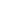A Survey of Gaussian Convolution Algorithms
Modules
Here is a list of all modules:Alvarez-Mazorra Gaussian convolution A first-order recursive filter approximation, computed in-placeBox filter Gaussian convolution A fast low-accuracy approximation of Gaussian convolutionDCT-based Gaussian convolution Convolution via multiplication in the DCT domainDeriche Gaussian convolution An accurate recursive filter approximation, computed out-of-placeExtended box filter Gaussian convolution An improvement of box filtering with continuous selection of sigmaFIR Gaussian convolution Approximation by finite impulse response filteringStacked integral images Gaussian convolution A sum of box filter responses, rather than a cascadeVliet-Young-Verbeek Gaussian convolution An accurate recursive filter approximation, computed in-place